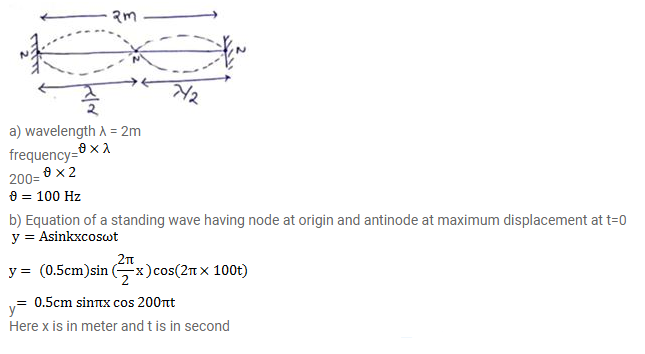# A 2m long string fixed at both ends is set into vibrations in its first overtone.Question:

A $2 \mathrm{~m}$ long string fixed at both ends is set into vibrations in its first overtone. The wave speed on the string is $200 \mathrm{~m} / \mathrm{s}$ and the amplitude is $0.5 \mathrm{~cm}$

a. Find the wavelength and frequency

b. Write the equation giving the displacement of different points as a function of time. Choose the $X$-axis along the string with the origin at one end and $t=0$ at the instant when the point $x=50 \mathrm{~cm}$ has reached its maximum displacement.

Solution: